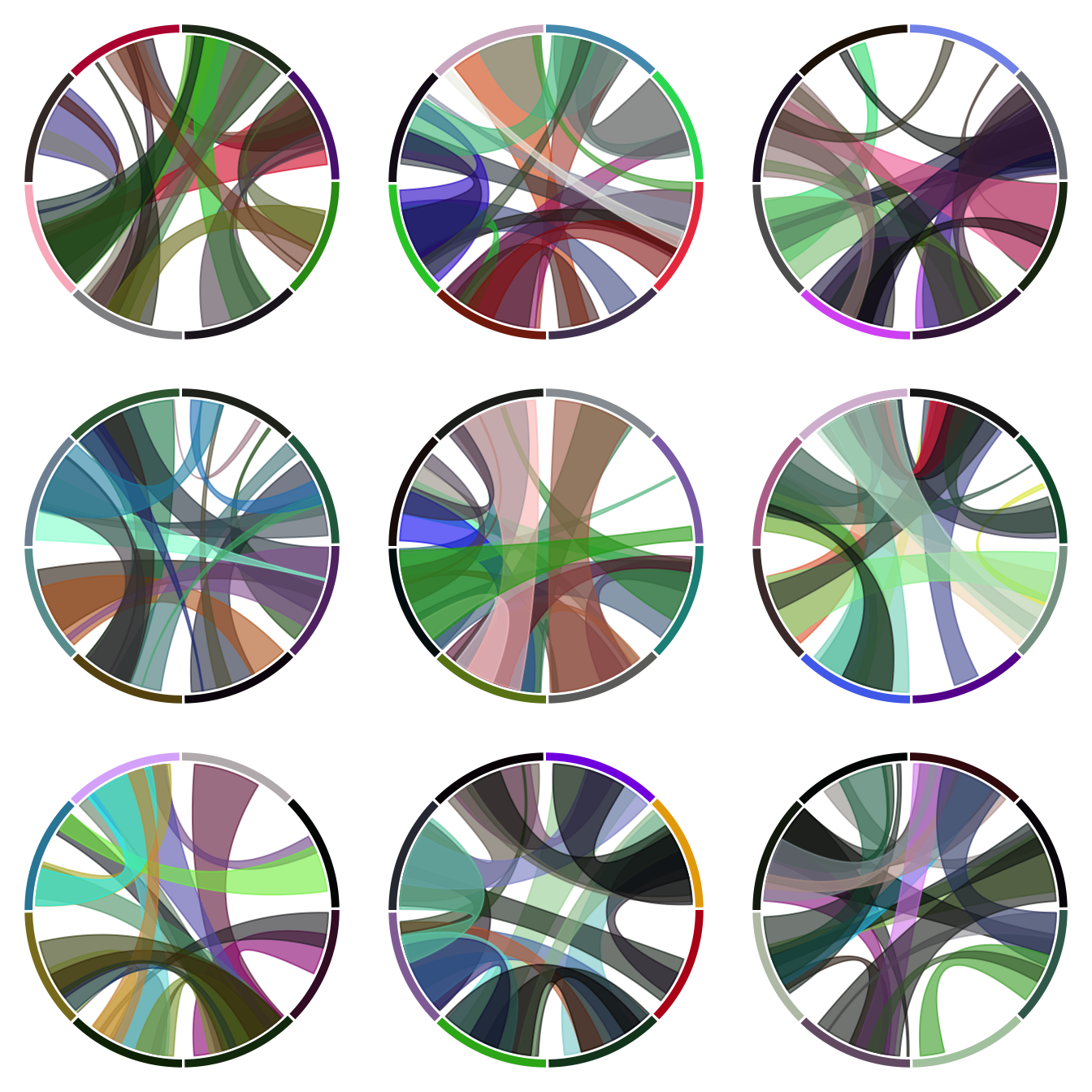# Display several chord diagrams on same figure

Here is a trick to display several chord diagrams on the same figure, using R and the circlize package.

To arrange several circular charts in the same layout, simply use the `layout()` function as shown below.

This example comes from the circlize package vignette.``````# library
library(circlize)
# Arrange the layout
layout(matrix(1:9, 3, 3))

# A loop to create 9 circular plots
for(i in 1:9) {
par(mar = c(0.5, 0.5, 0.5, 0.5), bg=rgb(1,1,1,0.1) )
factors = 1:8
circos.par(cell.padding = c(0, 0, 0, 0))
circos.initialize(factors, xlim = c(0, 1))
circos.trackPlotRegion(ylim = c(0, 1), track.height = 0.05, bg.col = rand_color(8), bg.border = NA )

for(i in 1:20) {
se = sample(1:8, 2)
circos.link(se, runif(2), se, runif(2), col = rand_color(1, transparency = 0.4))

}
circos.clear()
}``````

Related chart types

## Contact

This document is a work by Yan Holtz. Any feedback is highly encouraged. You can fill an issue on Github, drop me a message on Twitter, or send an email pasting yan.holtz.data with gmail.com.